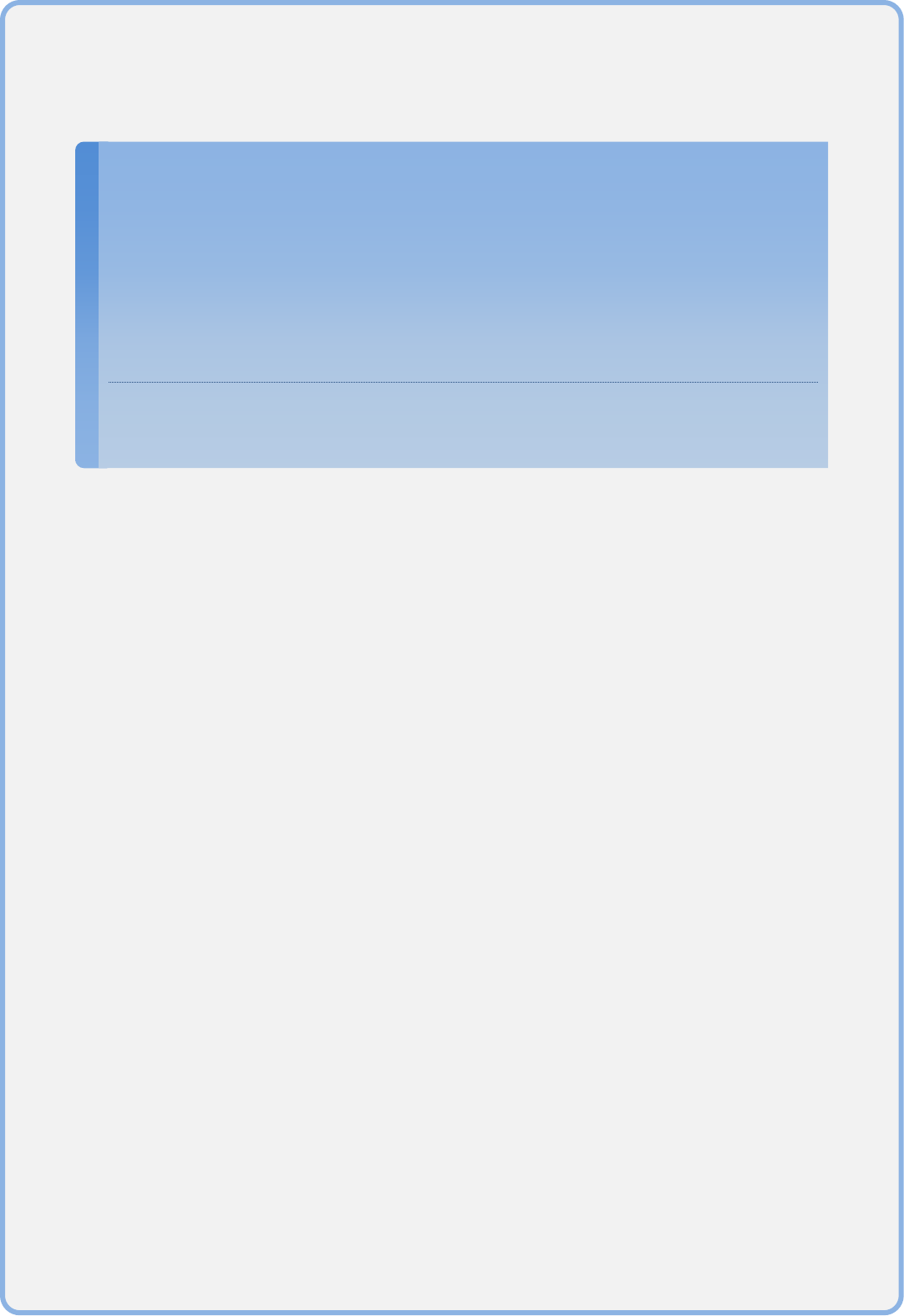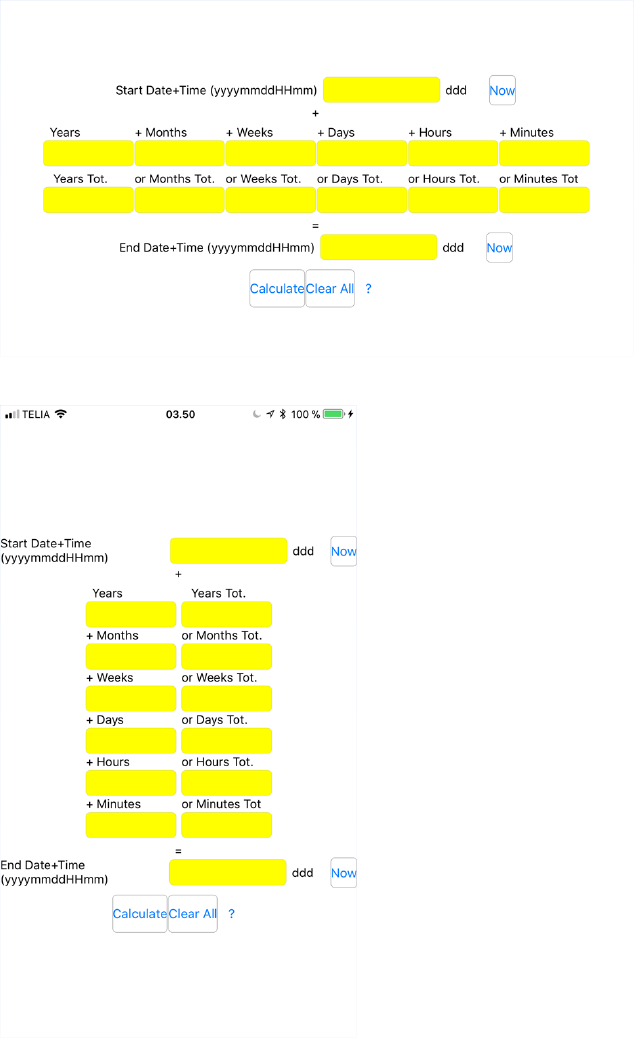TimeDateCalculator
User's guide
Rev. 1.1
Eigil Krogh Sorensen
TIMEDATECALCULATOR IN SHORT..................................................................3
SCREEN SHOTS. ..............................................................................................4
HOW IT WORKS..............................................................................................5
HOW TO USE IT. .............................................................................................6
TimeDateCalculator in short.
TimeDateCalculator calculates dates + times from time spans or time spans
from dates + times.
TimeDateCalculator runs on Windows 10, mac OS / X, iOS and Android.Screen shots.
Figure 1 Landscape screen shot
Figure 2 Portrait screen shot.
How it works.
As you can see from figure 1 and 2
There is a “Start Date+Time” and an “End Date+Time”.
There are Time Spans between the “Start Date+Time” and “End
Date+Time”:
o In the row/column with xxx “Tot” each box represents the total
number of whole Years, Months, Weeks, Days, Hours OR Minutes
between “Start Date+Time” and “End Date+Time”.
o In the row/column with “+” xxx the values in the boxes are added
together to give the full time span between “Start Date+Time” and
“End Date+Time”.
What TimeDateCalculator does is to “solve the equation” so the time
spans equals “End Date+Time” – “Start Date+Time”.
“ddd” will show the three first letters of the name of the day
corresponding to the Date+Time.How to use it.
You can use it in two ways:
1. Enter the “Start Date+Time” and “End Date+Time” and press calculate:
i. Then the time spans will be calculated.
2. Enter either
a. the “Start Date+Time” plus a time span and press calculate:
i. Then the “End Date+Time” will be calculated
OR
b. the “End Date+Time” plus a time span and press calculate:
i. Then the “Start Date+Time” will be calculated.
Have a good time!
Kind Regards
Eigil Krogh Sorensen